Next: Electromagnetic Momentum Conservation Up: Maxwell's Equations Previous: Retarded Fields

# Electromagnetic Energy Conservation

Consider the fourth Maxwell equation: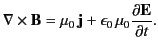(100)

Forming the scalar product with the electric field, and rearranging, we obtain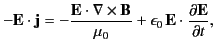(101)

which can be rewritten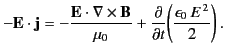(102)

Now,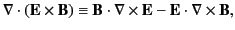(103)

so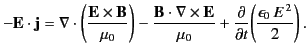(104)

Making use of third Maxwell equation,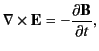(105)

we obtain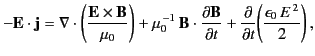(106)

which can be rewritten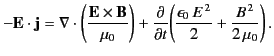(107)

Thus, we get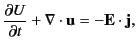(108)

where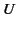and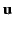are specified in Equations (109) and (110), respectively.

By comparison with Equation (7), we can recognize the previous expression as some sort of conservation equation. Here,is the density of the conserved quantity,is the flux of the conserved quantity, and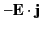is the rate at which the conserved quantity is created per unit volume. However,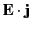is the rate per unit volume at which electric charges gain energy via interaction with electromagnetic fields. Hence,is the rate per unit volume at which electromagnetic fields gain energy via interaction with charges. It follows that Equation (108) is a conservation equation for electromagnetic energy. Thus.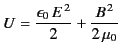(109)

can be interpreted as the electromagnetic energy density, and(110)

as the electromagnetic energy flux. The latter quantity is usually called the Poynting flux, after its discoverer.Next: Electromagnetic Momentum Conservation Up: Maxwell's Equations Previous: Retarded Fields
Richard Fitzpatrick 2014-06-27